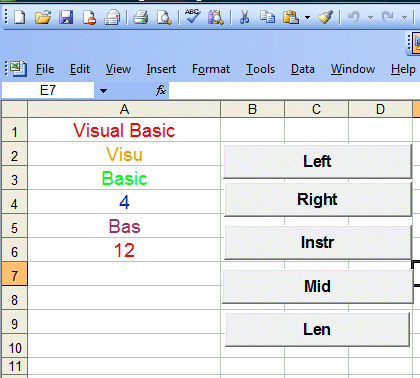# Lesson 8: The String Functions

Excel VBA uses various built-in functions to handle strings. These string handling functions are Left, Right, Instr, Mid and Len .  The following example illustrates the usage of all these functions.

#### 8.1 The InStr function

InStr is a function that looks for and returns the position of a substring in a phrase

##### Example 8.1

Private Sub cmdInstr_Click()
Dim phrase As String
phrase = Cells(1, 1).Value
Cells(4, 1) = InStr(phrase, "ual")
End Sub

The function InStr(phrase,"ual") will find the substring "ual" from the phrase "Visual Basic"  entered in cells(1,1) and then return its position, in this case, it is 4 from the left.

#### 8.2  The Left function

Left is a function that extracts the characters  from a phrase, starting from the left.

Left(phrase,4) means 4 characters are extracted from the phrase, starting from the leftmost position.

##### Example 8.2

Private Sub cmdLeft_Click()
Dim phrase As String
phrase = Cells(1, 1).Value
Cells(2, 1) = Left(phrase, 4)
End Sub

This code returns the substring "Visu" from the phrase "Visual Basic" entered in cells(1,1)

#### 8.3  The Right function

Right is a function that extracts characters  from a phrase, starting from the Right. Right(phrase,5) means 5 characters are extracted from the phrase, starting from the rightmost position.

##### Example 8.3

Private Sub cmdRight_Click()
Dim phrase As String
phrase = Cells(1, 1).Value
Cells(3, 1) = Right(phrase, 5)

This code returns the substring "Basic" from the phrase "Visual Basic" entered in cells(1,1)

#### 8.4  The Mid function

Mid is a function that extracts a substring  from a phrase, starting from the position specified by the second parameter in the bracket.

Mid(phrase,8,3) means a substring of three characters are extracted from the phrase, starting from the 8th position from the left, including empty space.

<
##### Example 8.4

Private Sub cmdMid_Click()
Dim phrase As String
phrase = Cells(1, 1).Value
Cells(5, 1) = Mid(phrase, 8, 3)
End Sub

This code returns the substring "Bas" from the phrase "Visual Basic" entered in cells(1,1)

#### 8.5  The Mid function

Len is a function that returns the length of  a phrase(including empty space in between)

##### Example 8.5

Private Sub cmdLen_Click()
Dim phrase As String
phrase = Cells(1, 1).Value
Cells(6, 1) = Len(phrase)
End Sub

The code returns 12 for the phrase "Visual Basic" entered in cells(1,1)

The output of all the examples are shown in Figure 8.1 below:Figure 8.1